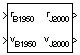# Besselian Epoch to Julian Epoch

Transform position and velocity components from discontinued Standard Besselian Epoch (B1950) to Standard Julian Epoch (J2000)

• Library:
• Aerospace Blockset / Utilities / Axes Transformations

•## Description

The Besselian Epoch to Julian Epoch block transforms two 3-by-1 vectors of Besselian Epoch position $\left({\overline{r}}_{B1950}\right)$, and Besselian Epoch velocity $\left({\overline{v}}_{B1950}\right)$ into Julian Epoch position $\left({\overline{r}}_{J2000}\right)$, and Julian Epoch velocity $\left({\overline{v}}_{J2000}\right)$. For more information on the transformation, see Algorithms.

## Ports

### Input

expand all

Position in Standard Besselian Epoch (B1950), specified as a 3-by-1 vector.

Data Types: `double`

Velocity in Standard Besselian Epoch (B1950), specified as a 3-by-1 vector.

Data Types: `double`

### Output

expand all

Position in Standard Julian Epoch (J2000), returned as a 3-by-1 vector.

Data Types: `double`

Velocity in Standard Julian Epoch (J2000), returned as a 3-by-1 vector.

Data Types: `double`

## Algorithms

The transformation is calculated using:

`$\left[\begin{array}{l}{\overline{r}}_{J2000}\\ {\overline{v}}_{J2000}\end{array}\right]=\left[\begin{array}{cc}{\overline{M}}_{rr}& {\overline{M}}_{vr}\\ {\overline{M}}_{rv}& {\overline{M}}_{vv}\end{array}\right]\left[\begin{array}{l}{\overline{r}}_{B1950}\\ {\overline{v}}_{B1950}\end{array}\right]$`

where $\left({\overline{M}}_{rr},{\overline{M}}_{vr},{\overline{M}}_{rv},{M}_{vv}\right)$ are defined as:

`${\overline{M}}_{rr}\left[\begin{array}{ccc}0.9999256782& -0.0111820611& -0.0048579477\\ 0.0111820610& 0.9999374784& -\text{0}\text{.0000271765}\\ 0.0048579479& -\text{0}\text{.0000271474}& 0.9999881997\end{array}\right]$`
`${\overline{M}}_{vr}=\left[\begin{array}{ccc}0.00000242395018& -\text{0}\text{.00000002710663}& -0.00000001177656\\ 0.00000002710663& 0.00000242397878& -0.00000000006587\\ 0.00000001177656& -0.00000000006582& 0.00000242410173\end{array}\right]$`
`${\overline{M}}_{rv}=\left[\begin{array}{ccc}-0.000551& -0.238565& 0.435739\\ 0.238514& -0.002667& -0.008541\\ -0.435623& 0.012254& 0.002117\end{array}\right]$`
`${\overline{M}}_{vv}=\left[\begin{array}{ccc}0.99994704& -0.01118251& -0.00485767\\ 0.01118251& 0.99995883& -0.00002718\\ 0.00485767& -0.00002714& 1.00000956\end{array}\right]$`

 "Supplement to Department of Defense World Geodetic System 1984 Technical Report: Part I - Methods, Techniques and Data Used in WGS84 Development," DMA TR8350.2-A.

## SupportGet trial now